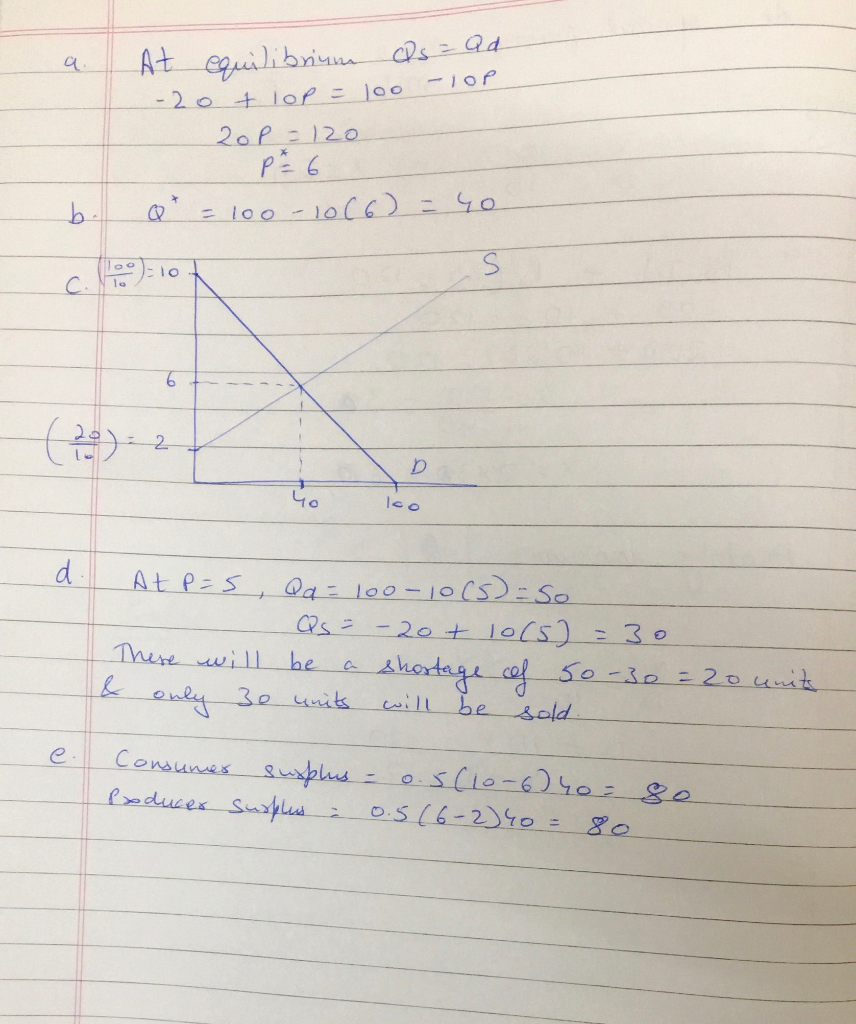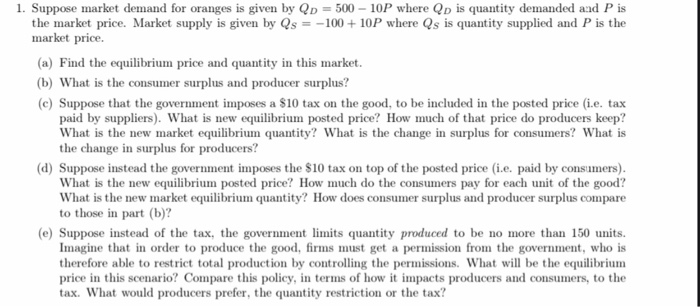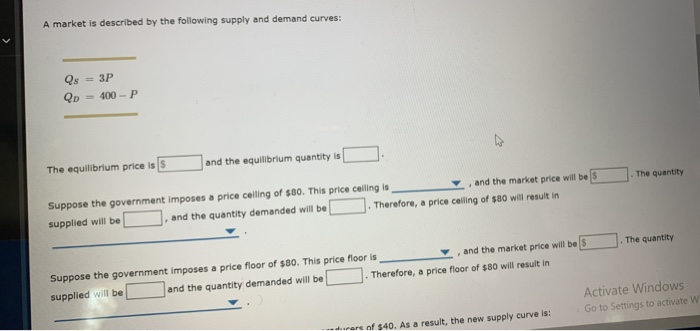Question

# Consider the market for oranges. The supply curve is QS=-20 +10P The demand curve is Qd...

1. Consider the market for oranges.

The supply curve is QS=-20 +10P

The demand curve is Qd =100-10P

1. Find the Equilibrium price.
2. Find the equilibrium quantity
3. Draw a rough sketch of your curves and depict the equilibrium
4. What will be the outcome if the government fixes the price at \$5.00 what will be the outcome?
5. Calculate the consumers’ and producers’ surplus at the equilibrium price.#### Earn Coins

Coins can be redeemed for fabulous gifts.

Similar Homework Help Questions
• ### 1. Suppose market demand for oranges is given by QD = 500 - 10P where Qp...1. Suppose market demand for oranges is given by QD = 500 - 10P where Qp is quantity demanded and P is the market price. Market supply is given by Qs = -100 + 10P where Qs is quantity supplied and P is the market price. (a) Find the equilibrium price and quantity in this market. (b) What is the consumer surplus and producer surplus? (C) Suppose that the government imposes a \$10 tax on the good, to be included...

• ### Assume: Demand Curve: QD = 80 – 10P; and Supply Curve: QS = 10P 7. Given...

Assume: Demand Curve: QD = 80 – 10P; and Supply Curve: QS = 10P 7. Given the information derived above, identify on the graph consumer surplus and producer surplus for each situation as well as deadweight loss, if any. d. Government imposes a sales tax of \$4.00, which is equally split between consumers and producers. What is the total surplus? Is the market operating efficiently? INCLUDE A GRAPH

• ### Suppose that demand and supply functions for good X are: QD=90-10P (P=9-0.1QD) QS=20P-6 (P=0.3+0.05QS) a. Graph...

Suppose that demand and supply functions for good X are: QD=90-10P (P=9-0.1QD) QS=20P-6 (P=0.3+0.05QS) a. Graph this situation. b. What is the equilibrium price and quantity in the market for good X? c. What is consumers surplus? Producers surplus? d. Suppose the government imposes a per unit tax on good X equal to 1 dollar (per unit). What is the new equilibrium price and quantity? How much revenue would this tax raise for the government? What is consumers surplus? Producers...

• ### Assume: Demand Curve: QD = 80 – 10P; and Supply Curve: QS = 10P 7. Given...

Assume: Demand Curve: QD = 80 – 10P; and Supply Curve: QS = 10P 7. Given the information derived above, identify on the graph consumer surplus and producer surplus for each situation as well as deadweight loss, if any. What is the total surplus? Quantify the quantity of goods imported. describe the implications of the imposition of a tariff in this market F. now assume a tariff of \$1.00 is added to the world price of \$2.00. INCLUDE A GRAPH

• ### Suppose that the demand curve for wheat is Qd= 400-10p Qs= 10p The government provides producers with a specific subsidy...

Suppose that the demand curve for wheat is Qd= 400-10p Qs= 10p The government provides producers with a specific subsidy of S=​\$11 per unit. How do the equilibrium price and quantity change? The equilibrium price by \$_______ and the equilibrium quantity by \$_______ units. (Enter numeric responses using real numbers rounded to two decimal places.) What effect does this tax (subsidy) have on consumer surplus, producer surplus, government revenue, welfare, and deadweight loss? Consumer surplus (increase or decrease) by \$...

• ### Assume: Demand Curve: QD = 80 – 10P; and Supply Curve: QS = 10P 7. Given...

Assume: Demand Curve: QD = 80 – 10P; and Supply Curve: QS = 10P 7. Given the information derived above, identify on the graph consumer surplus and producer surplus for each situation as well as deadweight loss, if any. b. Government imposes a minimum price of \$6.00 Calculate and assess (describe the impact) of the following: 1. Consumer Surplus 2. Producer Surplus 3. Deadweight Loss 4. Total Surplus 5. Government Revenue 6. Is the market operating efficiently: Yes or No....

• ### Assume: Demand Curve: QD = 80 – 10P; and Supply Curve: QS = 10P 7. Given...

Assume: Demand Curve: QD = 80 – 10P; and Supply Curve: QS = 10P 7. Given the information derived above, identify on the graph consumer surplus and producer surplus for each situation as well as deadweight loss, if any. INCLUDE A GRPAH c. Government imposes a maximum price of \$2.00. INCLUDE A GRAPH

• ### Assume: Demand Curve: QD = 80 – 10P; and Supply Curve: QS = 10P 7. Given...

Assume: Demand Curve: QD = 80 – 10P; and Supply Curve: QS = 10P 7. Given the information derived above, identify on the graph consumer surplus and producer surplus for each situation as well as deadweight loss, if any, total surplus and quantify the quantity of goods imported. e. now assume a world price of \$2.00. INCLUDE A GRAPH

• ### The supply and demand for 9-volt batteries are given by QD = 230 – 10P and...

The supply and demand for 9-volt batteries are given by QD = 230 – 10P and QS = 30P – 10, where P is the price per four-pack and Q measures the number of four-packs. a. What are the levels of consumer and producer surplus at the equilibrium price? b. Suppose that a hurricane causes widespread blackouts, shifting the demand curve for 9-volt batteries outward, with the new demand curve equal to QD = 690 – 10P. If the government...

• ### A market is described by the following supply and demand curves: Qs = 3P Qd =...A market is described by the following supply and demand curves: Qs = 3P Qd = 400-P The equilibrium price is S and the equilibrium quantity is Suppose the government imposes a price ceiling of \$80. This price ceiling is , and the market price will be supplied will be . and the quantity demanded will be . Therefore, a price calling of \$60 will result in the quantity the quantity Suppose the government imposes a price floor of \$80....